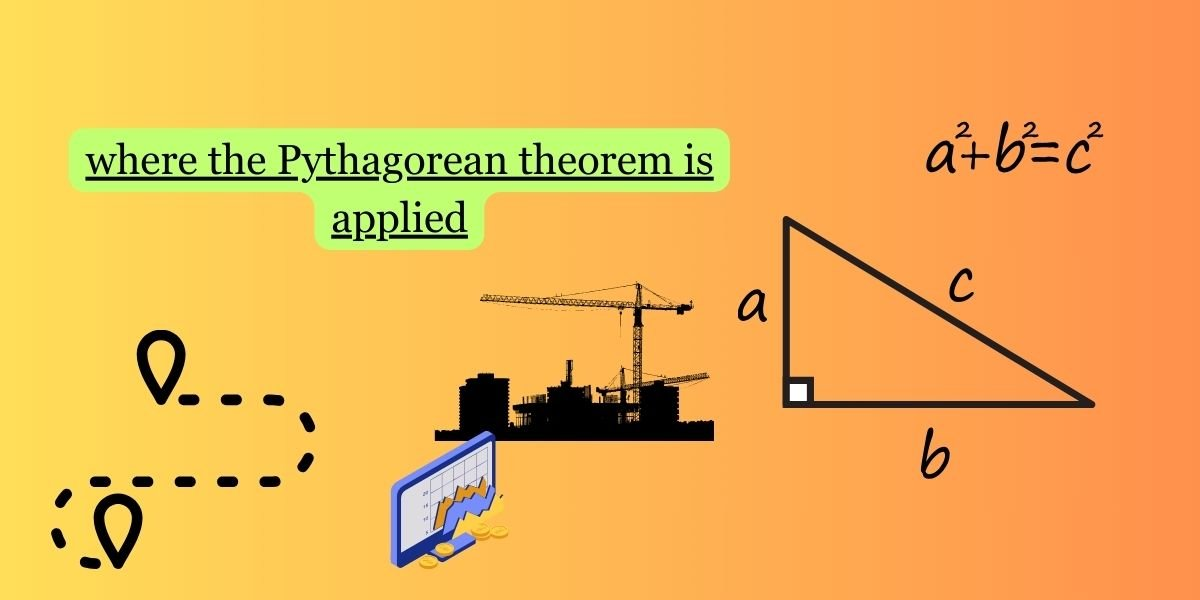Categories:

The Pythagorean Theorem, which states that in a right-angled triangle, the square of the length of the hypotenuse (the side opposite the right angle) is equal to the sum of the squares of the lengths of the other two sides, has numerous applications in various fields. Here are some practical applications where the Pythagorean Theorem is used:

1. Architecture and Construction:
• Architects use the Pythagorean Theorem to ensure that buildings and structures are stable and properly aligned.
• It helps in determining distances and angles, which is crucial in laying out the foundations and ensuring the correctness of the structure.
2. Surveying and Cartography:
• Surveyors use the theorem to measure distances and map out landscapes accurately.
• It’s essential in creating accurate maps and determining the boundaries of plots of land.
3. Engineering:
• Civil engineers use the Pythagorean Theorem in designing and constructing roads, bridges, and other infrastructure.
• Mechanical engineers use it in the design and analysis of machinery.
4. Physics:
• The theorem is used in physics for analyzing vectors and forces, especially in mechanics.
• It plays a role in calculating the trajectory of projectiles.
5. Computer Graphics and Gaming:
• In computer graphics, the Pythagorean Theorem is often used to calculate distances and positions of objects in a 2D or 3D space.
• Game developers use it for collision detection and character movement.
• Pilots and navigators use the theorem in aviation and maritime navigation to calculate distances and course corrections.
• GPS systems rely on mathematical principles, including the Pythagorean Theorem, to determine locations accurately.
7. Medical Imaging:
• In medical imaging, such as ultrasound and MRI, distances and angles within the body are calculated using the Pythagorean Theorem.
• It helps in determining the length of blood vessels, nerves, or other structures.
8. Trigonometry:
• The Pythagorean Theorem is fundamental to trigonometry, which has wide applications in science, engineering, and technology.
9. Finance:
• The theorem is used in financial mathematics for calculating distances and risks in investment portfolios.
10. Optics:
• Opticians use the theorem in lens design to calculate focal lengths and positions.

These are just a few examples, and the Pythagorean Theorem continues to be a cornerstone of geometry with widespread applications in various scientific, engineering, and mathematical fields.